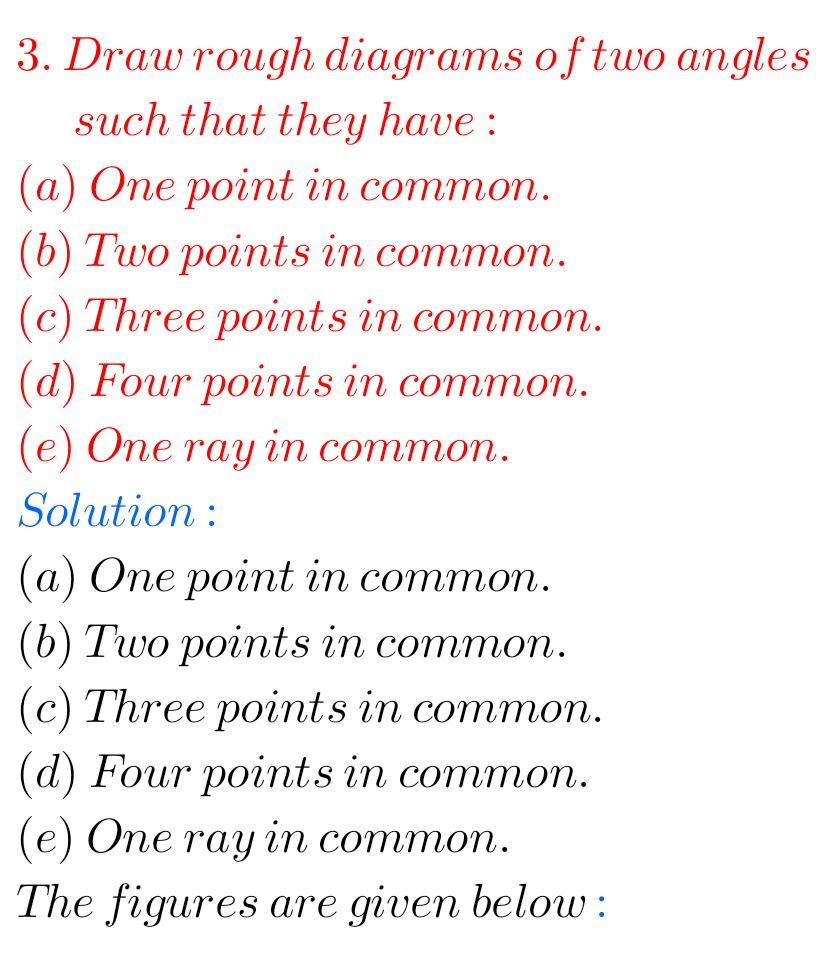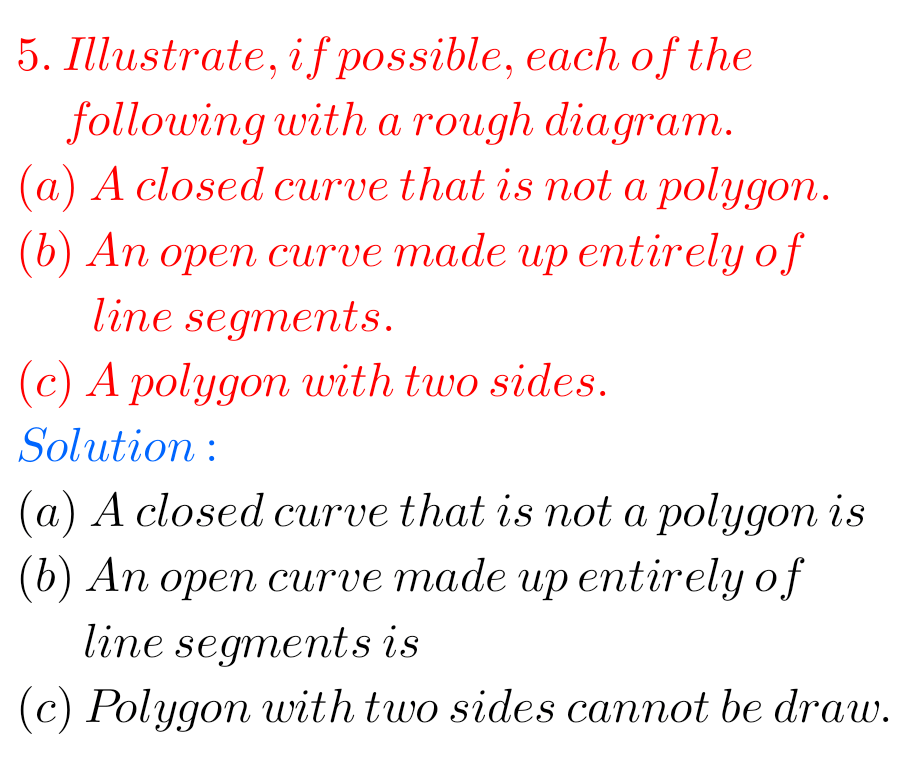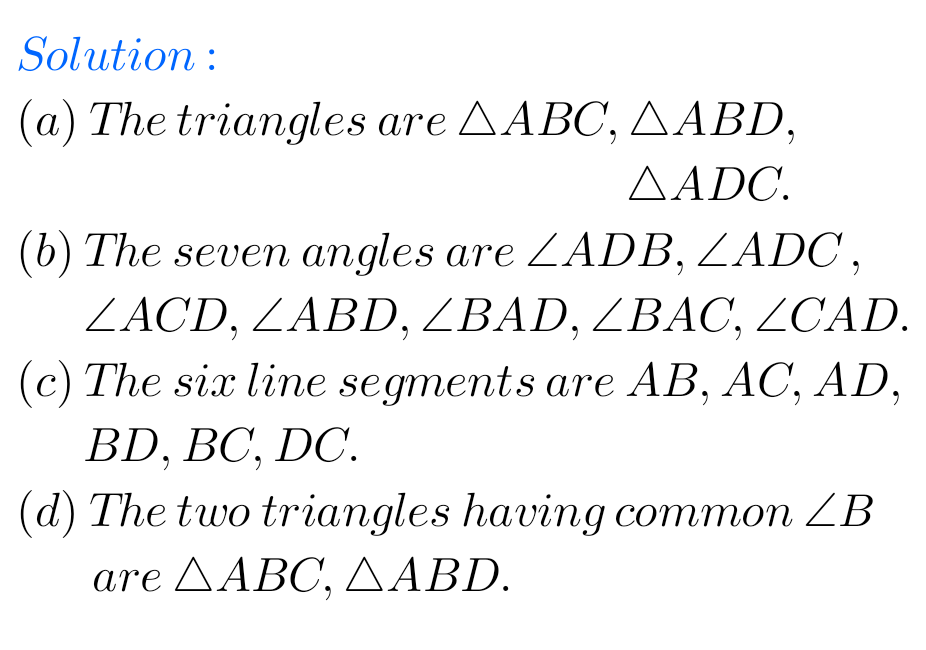# Chapter 4 Basic Geometrical Ideas Solutions class 6 Ncert## Basic Geometrical Ideas Solutions class 6 exercise 4.3 Ncert Maths

Basic Geometrical Ideas Solutions exercise 4.3 Class 6 NCERT Maths NCERT textbook Mathematics Class 6 Chapter 4 Basic Geometrical Ideas exercise 4.3 solutions are given. You should study the lesson Basic Geometrical Ideas in the textbook. You should observe the problems and solutions given in the textbook. Observe the given below problems and solutions and …## Maths Basic Geometrical Ideas Solutions exercise 4.2 class 6 Ncert

Basic Geometrical Ideas Solutions exercise 4.2 Class 6 NCERT Maths NCERT textbook Mathematics Class 6 Chapter 4 Basic Geometrical Ideas exercise 4.1 solutions are given. Study the lesson Basic Geometrical Ideas in the textbook. Observe the problems and solutions given in the textbook. Observe the given below problems and solutions and try them in your …## Ncert solutions for Maths Basic Geometrical Ideas Exercise 4.1 Class 6

Basic Geometrical Ideas Solutions exercise 4.1 Class 6 NCERT Maths NCERT textbook Mathematics Class 6 Chapter 4 Basic Geometrical Ideas exercise 4.1 solutions are given. You should study the lesson Basic Geometrical Ideas in the textbook. You should observe the problems and solutions given in the textbook. Observe the given below problems and solutions and …

## NCERT Maths Solutions for Class 6 Basic Geometrical Ideas Exercise 4.6

Basic Geometrical Ideas Exercise 4.6 Solutions Class 6 NCERT Ncert textbook Mathematics Class 6 Chapter 4 Basic Geometrical Ideas exercise 4.6 solutions are given. You should study the lesson Basic Geometrical Ideas in the textbook. You should also observe the example problems and solutions given in the textbook. Observe the solutions given below and try …## Solutions for Basic Geometrical Ideas Exercise 4.5 Maths Class 6 Ncert

Basic Geometrical Ideas Exercise 4.5 Solutions Class 6 Ncert Ncert textbook Mathematics Class 6 Chapter 4 Basic Geometrical Ideas exercise 4.5 solutions are given. Study the lesson Basic Geometrical Ideas in the textbook. Observe the problems and solutions given in the textbook. Observe the given below problems and solutions and try them in your own …## Basic Geometrical Ideas Solutions Class 6 Ncert Maths exercise 4.4

Maths Ncert Basic Geometrical Ideas Exercise 4.4 Solutions Ncert textbook Mathematics Class 6 Chapter 4 Basic Geometrical Ideas exercise 4.4 solutions are given. Study the lesson Basic Geometrical Ideas in the textbook. Observe the problems and solutions given in the textbook. Observe the given below problems and solutions and try them in your own method. …